Question

# Construct an amortization schedule for a \$1,000, 10% annual rate loan with 3 equal annual payments....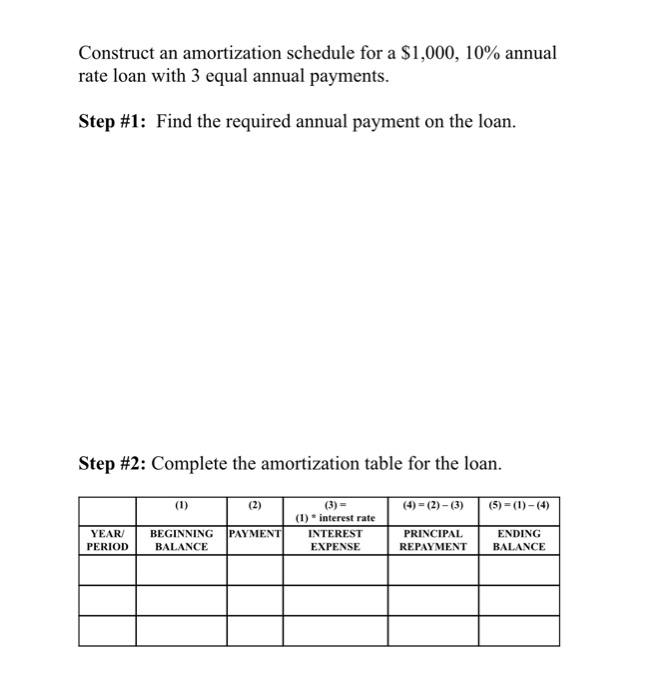Construct an amortization schedule for a \$1,000, 10% annual rate loan with 3 equal annual payments. Step #1: Find the required annual payment on the loan. Step #2: Complete the amortization table for the loan. (4) = (2)-(3) (5) = (1)-(4) (3) = (1) * interest rate INTEREST EXPENSE PAYMENT YEAR PERIOD BEGINNING BALANCE PRINCIPAL REPAYMENT ENDING BALANCE

SEE THE IMAGE. ANY DOUBTS, FEEL FREE TO ASK. THUMBS UP PLEASE

annual payment = 402.11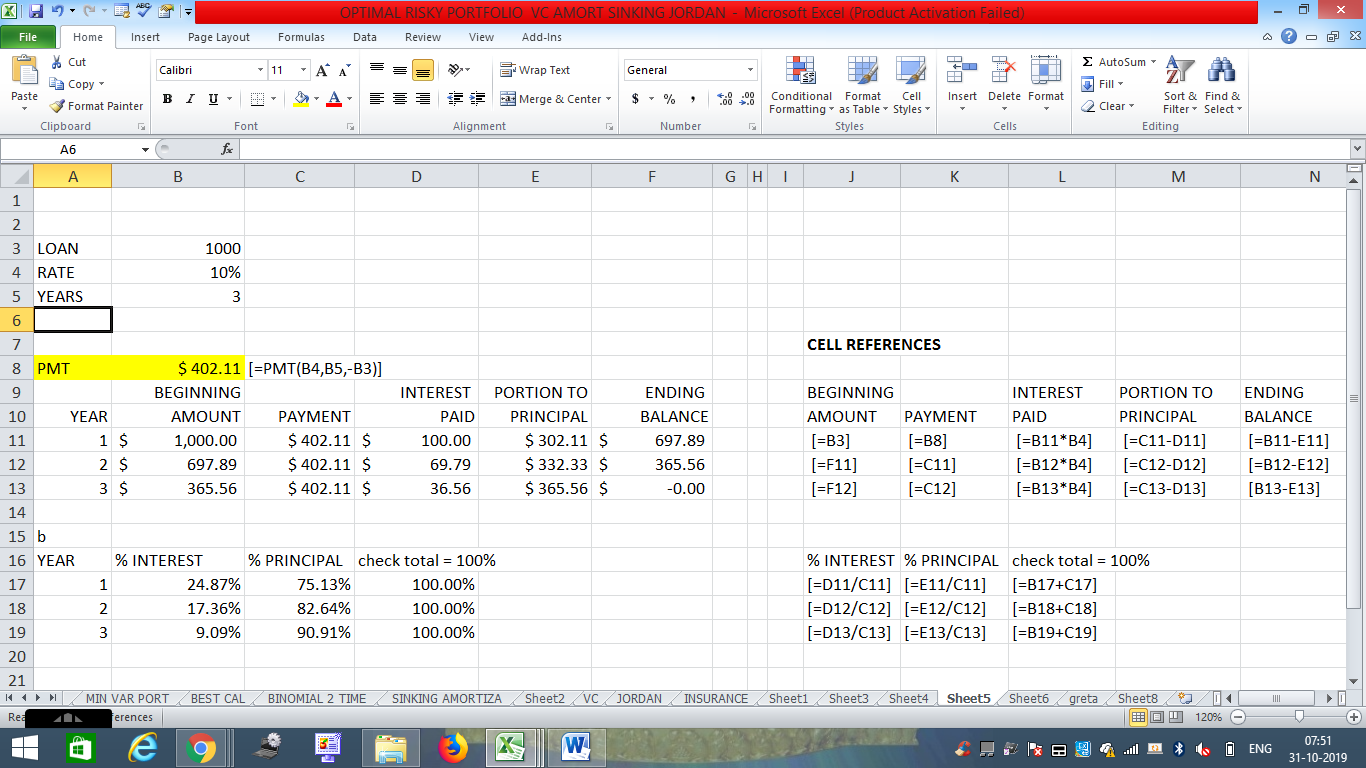#### Earn Coins

Coins can be redeemed for fabulous gifts.

Similar Homework Help Questions
• ### 8. Prepare the loan amortization schedule (\$15) You borrow \$1,000, and the loan is to be repaid in three equal paym...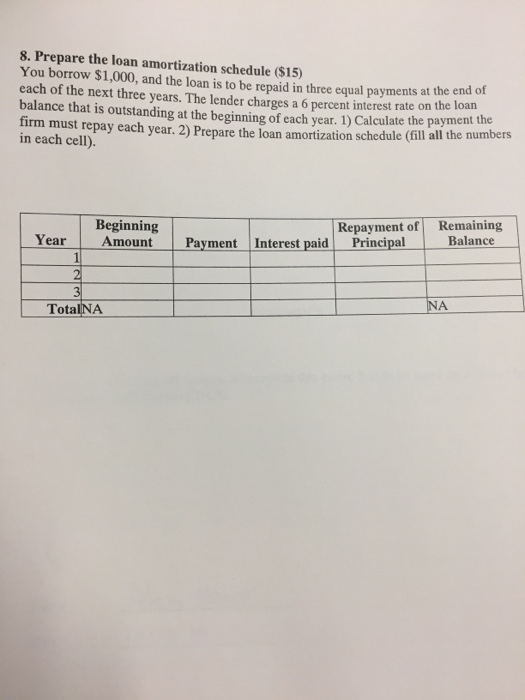8. Prepare the loan amortization schedule (\$15) You borrow \$1,000, and the loan is to be repaid in three equal payments at the end of each of the next three years. The lender charges a 6 percent interest rate on the loan balance that is outstanding at the beginning of each year. 1) Calculate the payment the firm must repay each year. 2) Prepare the loan amortization schedule (fill all the numbers in each cell). Repayment of Remaining Principal Beginning...

• ### 8. Prepare the loan amortization schedule (\$15) You borrow \$1,000, and the loan is to be repaid in three equal paym...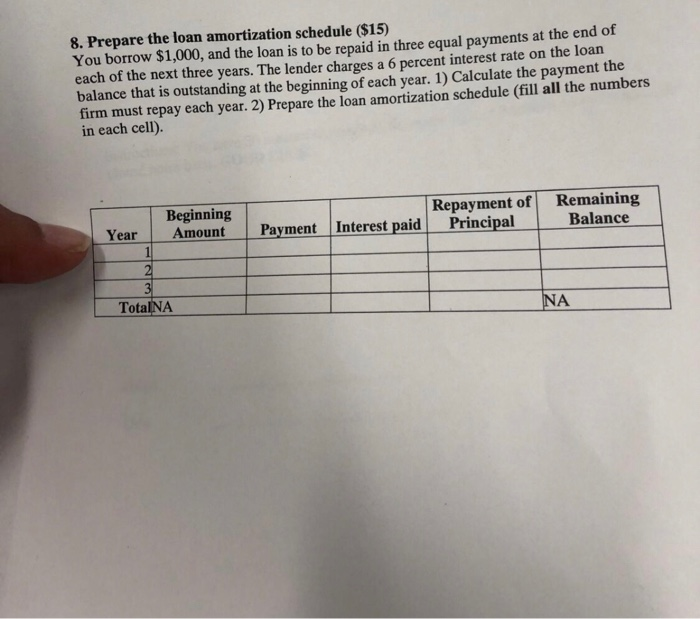8. Prepare the loan amortization schedule (\$15) You borrow \$1,000, and the loan is to be repaid in three equal payments at the end of each of the next three years. The lender charges a 6 percent interest rate on the loan balance that is outstanding at the beginning of each year. 1) Calculate the payment the firm must repay each year. 2) Prepare the loan amortization schedule (fill all the numbers in each cell). Beginning Amount Repayment of Principal...

• ### 8. Prepare the loan amortization schedule (\$15) You borrow \$1,000, and the loan is to be...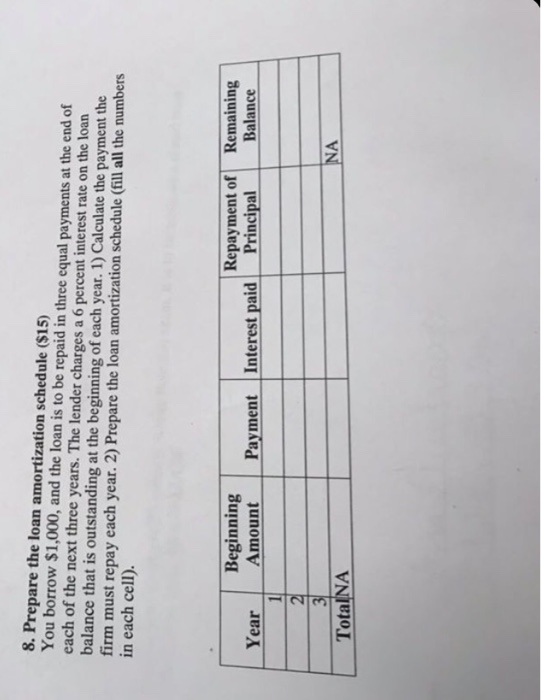8. Prepare the loan amortization schedule (\$15) You borrow \$1,000, and the loan is to be repaid in three equal payments at the end of each of the next three years. The lender charges a 6 percent interest rate on the loan balance that is outstanding at the beginning of each year. 1) Calculate the payment the firm must repay each year. 2) Prepare the loan amortization schedule (fill all the numbers in each cell). Beginning Amount Repayment of Remaining...

• ### Loan amortization schedule Personal Finance Problem Joan Messineo borrowed \$46,000 at a 4% annual rate of...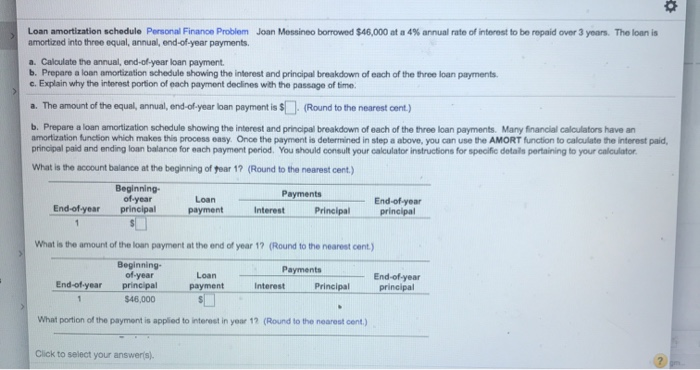Loan amortization schedule Personal Finance Problem Joan Messineo borrowed \$46,000 at a 4% annual rate of interest to be repaid over 3 years. The loan is amortized into three equal, annual end-of-year payments Calculate the annual end of year loan payment b. Prepare a loan amortization schedule showing the interest and principal breakdown of each of the three loan payments. c. Explain why the interest portion of each payment declines with the passage of time. a. The amount of the...

• ### Loan amortization schedule  Personal Finance Problem   Joan Messineo borrowed ​\$49 comma 00049,000 at a 66​% annual...

Loan amortization schedule  Personal Finance Problem   Joan Messineo borrowed ​\$49 comma 00049,000 at a 66​% annual rate of interest to be repaid over 3 years. The loan is amortized into three​ equal, annual,​ end-of-year payments. a.  Calculate the​ annual, end-of-year loan payment. b.  Prepare a loan amortization schedule showing the interest and principal breakdown of each of the three loan payments. c. Explain why the interest portion of each payment declines with the passage of time. a.  The amount of...

• ### AMORTIZATION SCHEDULE a. Complete an amortization schedule for a \$34,000 loan to be repaid in equal...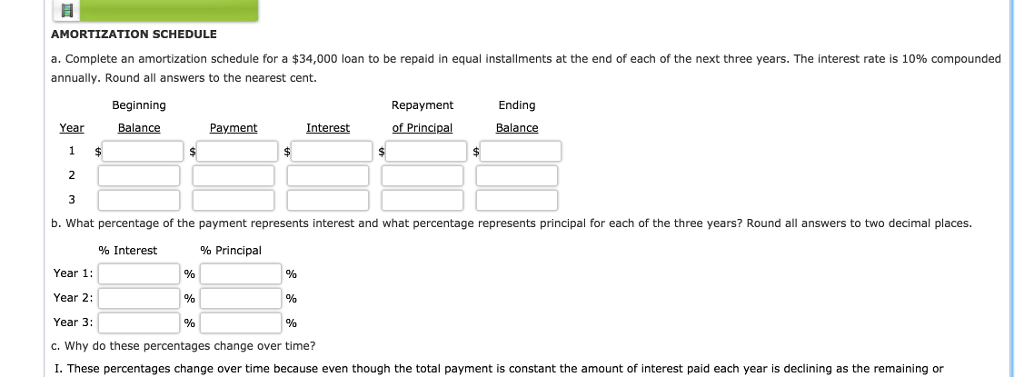AMORTIZATION SCHEDULE a. Complete an amortization schedule for a \$34,000 loan to be repaid in equal installments at the end of each of the next three years. The interest rate is 10% compounded annually. Round all answers to the nearest cent. Beginning Repayment Ending Interest ofPrincipal Balance Balance avment Interest Payment b. What percentage of the payment represents interest and what percentage represents principal for each of the three years? Round all answers to two decimal places. 96 Interest %...

• ### Given the annual interest rate and a line of an amortization schedule for that loan, complete...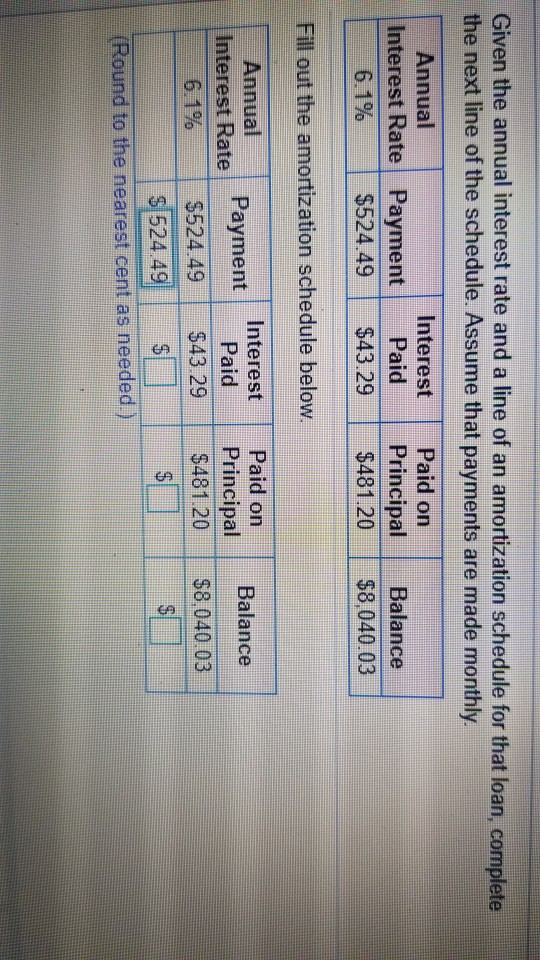Given the annual interest rate and a line of an amortization schedule for that loan, complete the next line of the schedule. Assume that payments are made monthly. Annual Interest Rate Payment \$524.49 Interest Paid \$43.29 Paid on Principal \$481.20 Balance \$8,040.03 out the amortization schedule below. Payment Balance Annual interest Rate 6.1% Interest Paid \$43.29 Paid on Principal \$481.20 \$8.040.03 \$524.49 \$ 524.49 Round to the nearest cent as needed.

• ### wity. Amortization schedule Excel Online Structured Activity: Amortization schedule The data on a loan has been...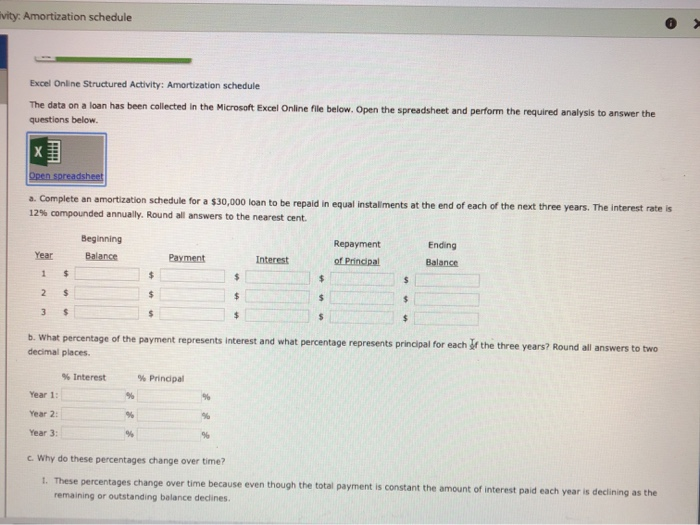wity. Amortization schedule Excel Online Structured Activity: Amortization schedule The data on a loan has been collected in the Microsoft Excel Online file below. Open the spreadsheet and perform the required analysis to answer the questions below. X Open spreadshee a. Complete an amortization schedule for a \$30,000 loan to be repaid in equal installments at the end of each of the next three years. The interest rate is 12% compounded annually. Round all answers to the nearest cent Ending...

• ### Prepare an amortization schedule for a three-year loan of \$15,000. The interest rate is 10 percent...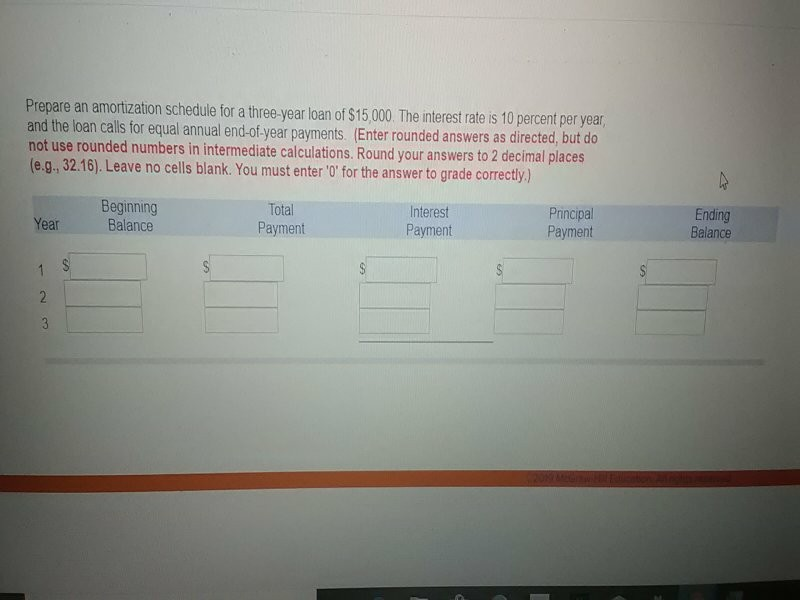Prepare an amortization schedule for a three-year loan of \$15,000. The interest rate is 10 percent per year and the loan calls for equal annual end-of-year payments (Enter rounded answers as directed, but do not use rounded numbers in intermediate calculations. Round your answers to 2 decimal places (e.g., 32.16). Leave no cells blank. You must enter '0' for the answer to grade correctly) Beginning Balance Total Interest Principal Payment Ending Balance Year Payment Payment 1 S 2 3

• ### Suppose C&Y restaurant borrow a 5-year loan of \$85,000 at an annual interest rate of 5%....

Suppose C&Y restaurant borrow a 5-year loan of \$85,000 at an annual interest rate of 5%. The loan agreement states that the repayment of principal and the loan interest has to be paid by the end of each year. The instalment of each repayment is fixed amount throughout the loan period. You are instructed to construct an amortization schedule for loan repayment including beginning balance, annual payment, interest and ending balance.